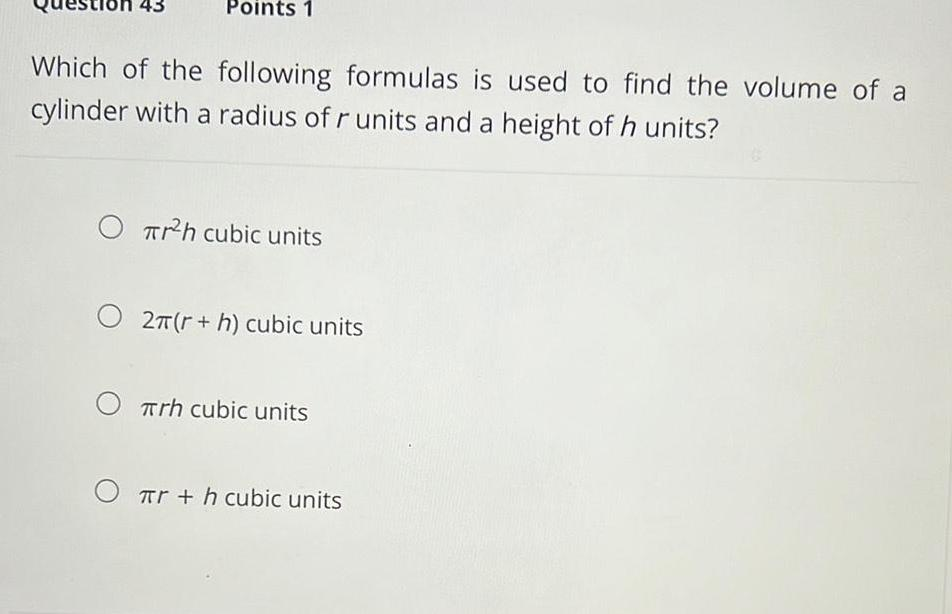Question:

# Points 1 Which of the following formulas is used to find the

Last updated: 8/2/2023Points 1 Which of the following formulas is used to find the volume of a cylinder with a radius of r units and a height of h units O Trh cubic units O 2 r h cubic units Trh cubic units r h cubic units